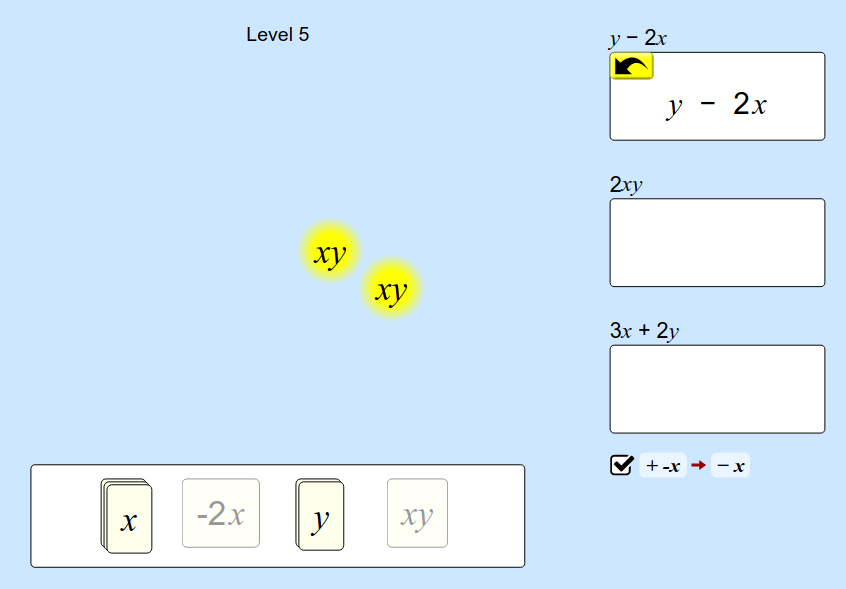MM Practice
×
Multiplication
Division
Place Value
Fractions & Decimals
Measurement
Statistics & Probability
Pre-Algebra
Money
Kindergarten
Geometry

# From terms to algebraic expressions: Expression Exchange GameOnline game for 6th-9th grade math

This online activity includes THREE separate work areas ("Basics", "Explore", and "Negatives") where you can explore how simple algebraic expressions work, and then one game.

In the work areas, you can learn how to add and subtract simple algebraic terms in order to form an expression. For example, you can put together x, xy, another x, and −1 to form 2x + xy −1.

• To add two terms, drag one term near the other. They will "snap" together and a PLUS sign is added between them
• To add similar terms, drag one ON TOP of the other. For example, if you drag y on top of another y, they will transform to −2y.
• to subtract terms, you will need to find a negative form of the term in the list, such as −y, and then add it (drag it near another term).
• To simplify an expression that has similar terms, you need to first cut the expression. Click the term you want to combine with another term. A "scissors" button appears; click it. After that, the terms are separate and you can drag one on top of another to combine them (only similar terms, of course!).

The game is where you are asked to build certain expressions from the "parts" (given terms).

These activities will help students to understand how simple algebraic expressions (polynomials) work: how terms are added, how coefficients work, and how the distributive property is applied. It fits best pre-algebra and algebra 1 courses; however, the "Basics" workarea is usable even for 6th grade math work.

Credit: This activity is created by PhET

Screenshot from the game:How to combine expresssions:

Allow my comment to be posted on this site